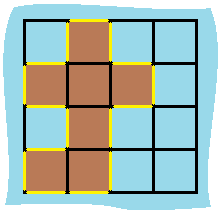## Algorithm

Problem Name: 463. Island Perimeter

You are given `row x col` `grid` representing a map where `grid[i][j] = 1` represents land and `grid[i][j] = 0` represents water.

Grid cells are connected horizontally/vertically (not diagonally). The `grid` is completely surrounded by water, and there is exactly one island (i.e., one or more connected land cells).

The island doesn't have "lakes", meaning the water inside isn't connected to the water around the island. One cell is a square with side length 1. The grid is rectangular, width and height don't exceed 100. Determine the perimeter of the island.

Example 1:```Input: grid = [[0,1,0,0],[1,1,1,0],[0,1,0,0],[1,1,0,0]]
Output: 16
Explanation: The perimeter is the 16 yellow stripes in the image above.
```

Example 2:

```Input: grid = []
Output: 4
```

Example 3:

```Input: grid = [[1,0]]
Output: 4
```

Constraints:

• `row == grid.length`
• `col == grid[i].length`
• `1 <= row, col <= 100`
• `grid[i][j]` is `0` or `1`.
• There is exactly one island in `grid`.

## Code Examples

### #1 Code Example with C Programming

```Code - C Programming```

``````
int islandPerimeter(int** grid, int gridRowSize, int gridColSize) {
int m = 0;
int i, j;
for (i = 0; i < gridRowSize; i ++) {
for (j = 0; j < gridColSize; j ++) {
if (grid[i][j] == 1) {
if (i == 0 || grid[i - 1][j] == 0) {
m ++;
}
if (i == gridRowSize - 1 || grid[i + 1][j] == 0) {
m ++;
}
if (j == 0 || grid[i][j - 1] == 0) {
m ++;
}
if (j == gridColSize - 1 || grid[i][j + 1] == 0) {
m ++;
}
}
}
}
return m;
}
``````
Copy The Code &

Input

cmd
grid = [[0,1,0,0],[1,1,1,0],[0,1,0,0],[1,1,0,0]]

Output

cmd
16

### #2 Code Example with Java Programming

```Code - Java Programming```

``````
class Solution {
int[][] dirs = {{1, 0}, {0, 1}, {-1, 0}, {0, -1}};
public int islandPerimeter(int[][] grid) {
int sum = 0;
for (int i = 0; i < grid.length; i++) {
for (int j = 0; j < grid.length; j++) {
if (grid[i][j] == 1) {
for (int[] dir : dirs) {
sum += isBoundary(grid, i + dir, j + dir) ? 1 : 0;
}
}
}
}
return sum;
}

private boolean isBoundary(int[][] grid, int x, int y) {
return x < 0 || x >= grid.length || y < 0 || y >= grid.length || grid[x][y] == 0;
}
}
``````
Copy The Code &

Input

cmd
grid = [[0,1,0,0],[1,1,1,0],[0,1,0,0],[1,1,0,0]]

Output

cmd
16

### #3 Code Example with Javascript Programming

```Code - Javascript Programming```

``````
const islandPerimeter = function(grid) {
let len = 0;
for (let r = 0; r < grid.length; r++) {
for (let c = 0; c < grid.length; c++) {
if (grid[r][c] === 1) {
len += cell(grid, r, c);
}
}
}
return len;
};

function cell(grid, r, c) {
let len = 0;
// top
if (r === 0 || grid[r - 1][c] !== 1) {
len += 1;
}
// left
if (c === 0 || grid[r][c - 1] !== 1) {
len += 1;
}
// right
if (grid[r][c + 1] !== 1) {
len += 1;
}
// bottom
if (grid[r + 1] == null || grid[r + 1][c] !== 1) {
len += 1;
}
return len;
}

console.log(
islandPerimeter([[0, 1, 0, 0], [1, 1, 1, 0], [0, 1, 0, 0], [1, 1, 0, 0]])
);
``````
Copy The Code &

Input

cmd
grid = []

Output

cmd
4

### #4 Code Example with Python Programming

```Code - Python Programming```

``````
class Solution:
def islandPerimeter(self, grid: List[List[int]]) -> int:
self.res = 0
used = set()
def dfs(i, j):
self.res += 4
for x, y in (i - 1, j), (i + 1, j), (i, j - 1), (i, j + 1):
if 0 <= x < m and 0 <= y < n and grid[x][y]:
self.res -= 1
if (x, y) not in used:
dfs(x, y)
m, n = len(grid), len(grid)
for i in range(m):
for j in range(n):
if grid[i][j] == 1 and (i, j) not in used:
dfs(i, j)
return self.res
``````
Copy The Code &

Input

cmd
grid = []

Output

cmd
4

### #5 Code Example with C# Programming

```Code - C# Programming```

``````
namespace LeetCode
{
public class _0463_IslandPerimeter
{
public int IslandPerimeter(int[][] grid)
{
var result = 0;

var row = grid.Length;
var col = grid.Length;
for (int i = 0; i < row; i++)
for (int j = 0; j < col; j++)
{
if (grid[i][j] == 1)
{
result += 4;
if (i > 0 && grid[i - 1][j] == 1)
result -= 2;
if (j > 0 && grid[i][j - 1] == 1)
result -= 2;
}
}

return result;
}
}
}
``````
Copy The Code &

Input

cmd
grid = [[1,0]]

Output

cmd
4